# 日拱一卒，伯克利CS61A完结撒花### Q1: Compose All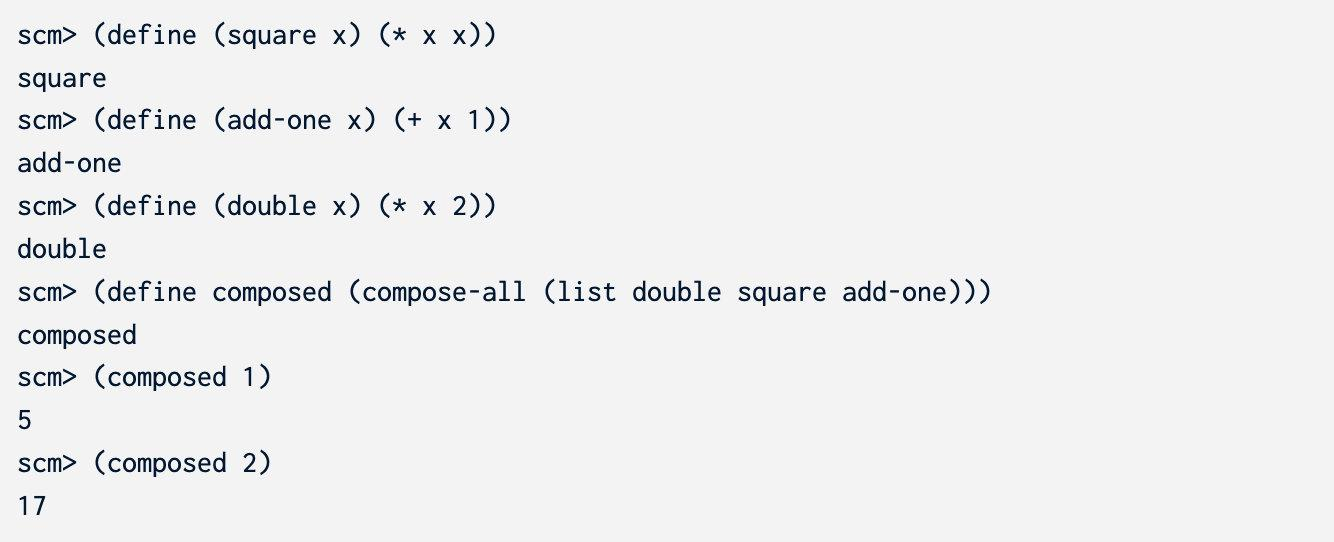```scheme (define (compose-all funcs) 'YOUR-CODE-HERE nil )```

#### 答案

```scheme (define (compose-all funcs) (define (dfs funcs x) (if (null? funcs) x (dfs (cdr funcs) ((car funcs) x)) ) ) (lambda (x) (dfs funcs x) ) )```

## Tail Recursion

### Q2: Replicate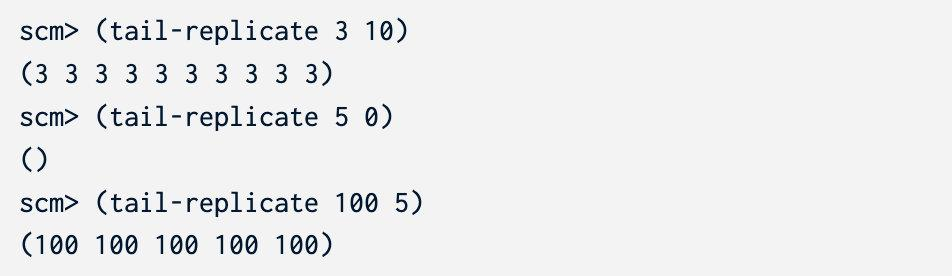#### 答案

```scheme (define (tail-replicate x n) (define (dfs x n ret) (if (= n 0) ret (dfs x (- n 1) (cons x ret)) ) ) (dfs x n nil) )```

## Generators

```python def permutations(lst): """Generates all permutations of sequence LST. Each permutation is a list of the elements in LST in a different order.

``````The order of the permutations does not matter.

>>> sorted(permutations([1, 2, 3]))
[[1, 2, 3], [1, 3, 2], [2, 1, 3], [2, 3, 1], [3, 1, 2], [3, 2, 1]]
>>> type(permutations([1, 2, 3]))
<class 'generator'>
>>> sorted(permutations((10, 20, 30)))
[[10, 20, 30], [10, 30, 20], [20, 10, 30], [20, 30, 10], [30, 10, 20], [30, 20, 10]]
>>> sorted(permutations("ab"))
[['a', 'b'], ['b', 'a']]
"""
if not lst:
yield []
return
``````

```

#### 答案

```python def permutations(lst): if not lst: yield [] return "*** YOUR CODE HERE ***" if len(lst) == 1: yield lst return v = lst[-1] for p in permutations(lst[:-1]): for i in range(0, len(p)+1): yield list(p[:i]) + [v] + list(p[i: ])```

# Optional Questions

## Streams

### Q4: Run-Length Encoding

Run-length encoding是一个非常简单的数据压缩技术，这项技术把相同的数压缩成一个数。一个相同的数字序列被称为一个run。比如下面这个有限序列：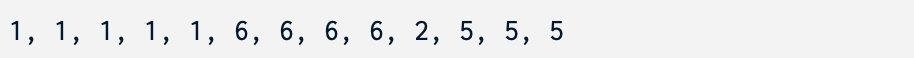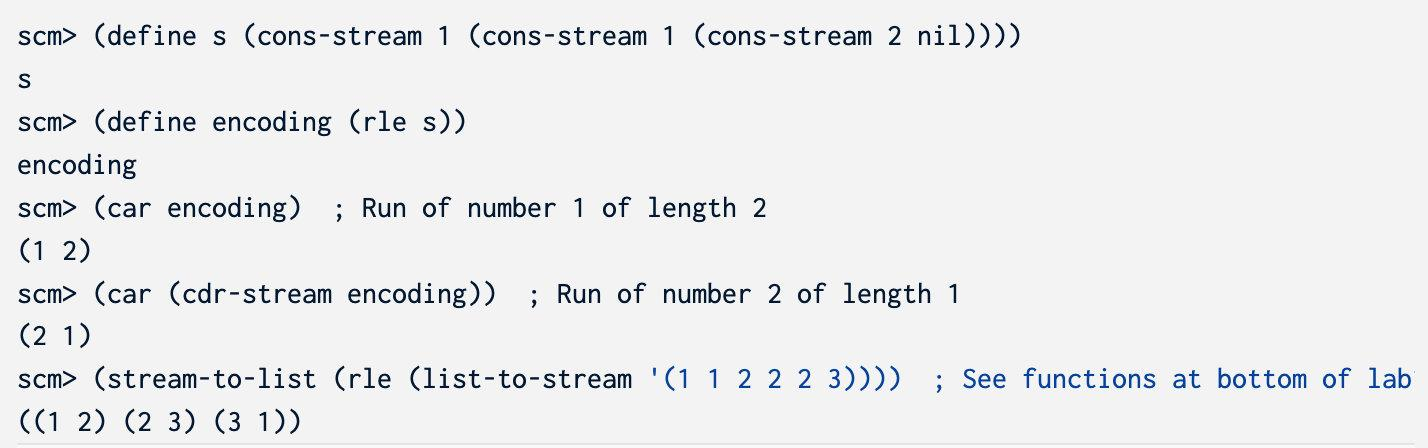#### 答案

```scheme (define (rle s) (define (helper v cnt s) (cond ((null? s) (cons-stream (list v cnt) nil)) ((equal? v (car s)) (helper v (+ cnt 1) (cdr-stream s))) (else (cons-stream (list v cnt) (helper (car s) 1 (cdr-stream s)))) ) ) (if (null? s) nil (helper (car s) 1 (cdr-stream s))) )```

## More Tail Recursion

### Q5: Insert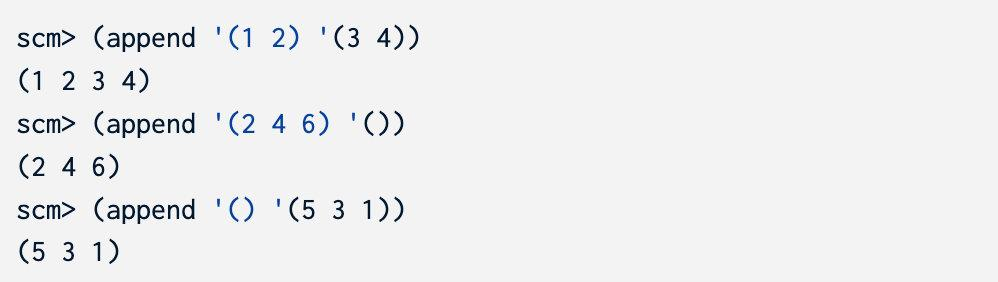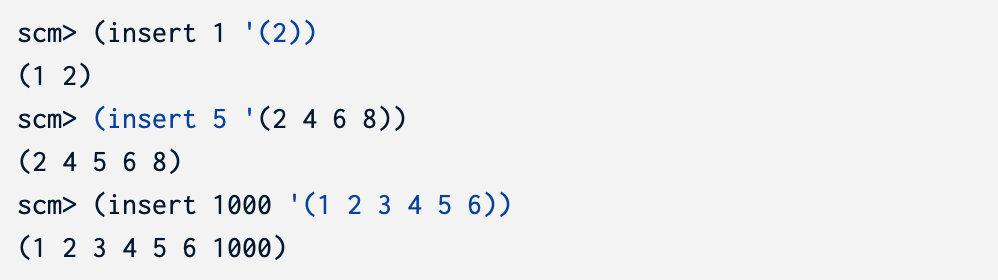ok测试只能检查你的结果是否准确，不能检查你是否使用了尾递归。你可以使用一些人工的大测试样例来检查比如：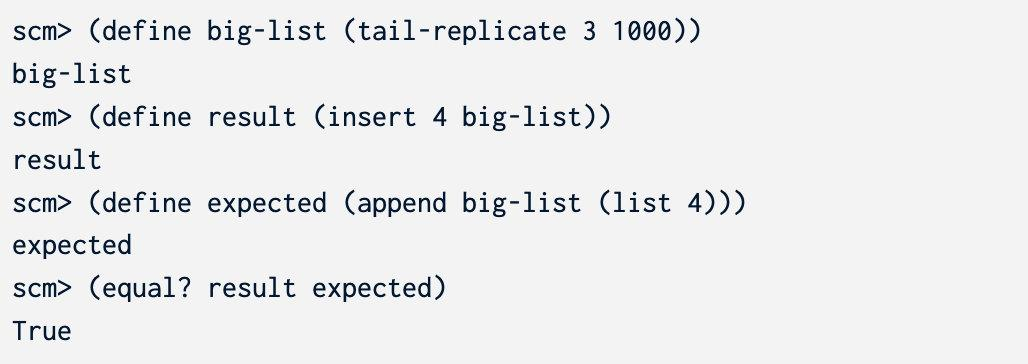#### 答案

```scheme (define (insert n s) (define (helper n prev suf) (if (null? suf) (append prev (list n)) (if (< n (car suf)) (append (if (null? prev) (list n) (append prev (list n))) suf) (helper n (append prev (list (car suf))) (cdr suf)) ) ) ) (helper n nil s) )```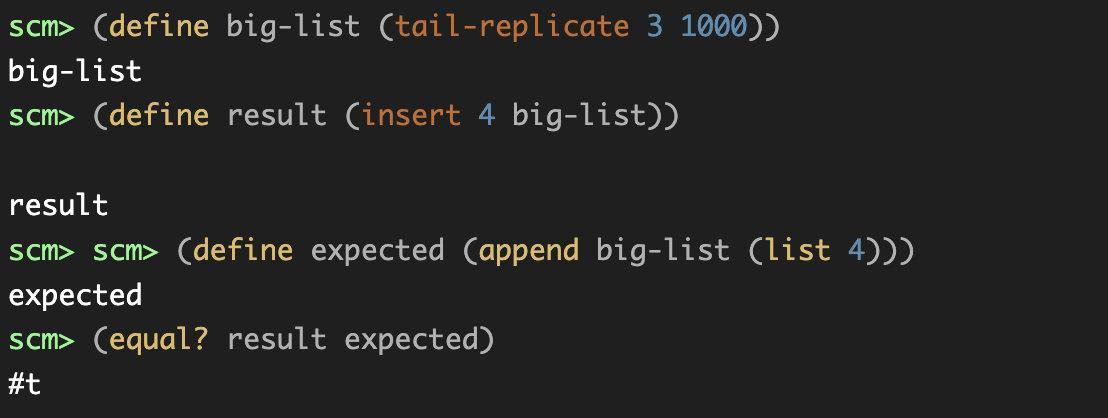### Q6: Deep Map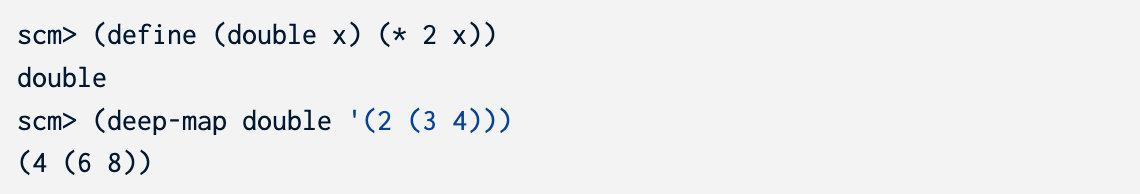#### 答案

```scheme (define (deep-map fn s) (if (null? s) nil (if (list? (car s)) (cons (deep-map fn (car s)) (deep-map fn (cdr s))) (cons (fn (car s)) (deep-map fn (cdr s))) ) ) )```

### Q7: Tally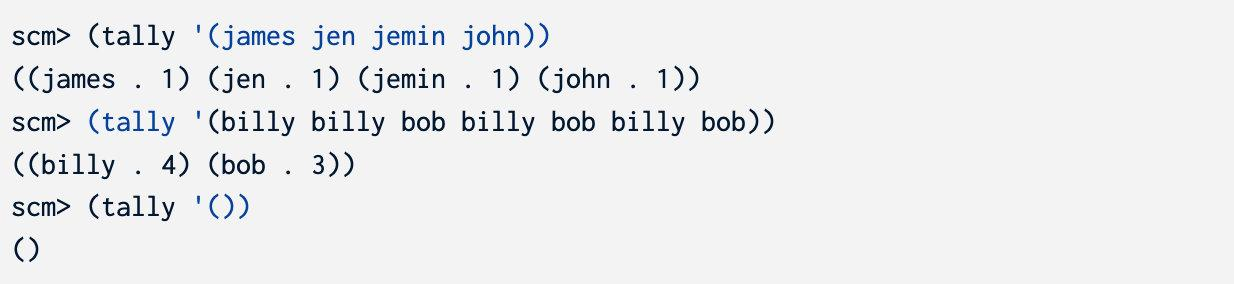#### 答案

`count`非常简单，就是一个递归的简单使用。

`unique`如果我们自己实现也会非常麻烦，使用`filter`之后会变得简单很多。

```scheme (define (map fn s) (if (null? s) nil (cons (fn (car s)) (map fn (cdr s)))))

(define (filter fn s) (cond ((null? s) nil) ((fn (car s)) (cons (car s) (filter fn (cdr s)))) (else (filter fn (cdr s)))))

; Implementing and using these helper procedures is optional. You are allowed ; to delete them. (define (unique s) (if (null? s) nil (cons (car s) (filter (lambda (x) (not (eq? (car s) x))) (unique (cdr s)))) ) )

(define (count name s) (if (null? s) 0 (if (eq? name (car s)) (+ 1 (count name (cdr s))) (count name (cdr s)) ) ) )

(define (tally names) (map (lambda (x) (cons x (count x names))) (unique names)) ) ```

## More Generators

### Q8: Generators generator

```python def make_generators_generator(g): """Generates all the "sub"-generators of the generator returned by the generator function g.

``````>>> def ints_to(n):
...     for i in range(1, n + 1):
...          yield i
...
>>> def ints_to_5():
...     for item in ints_to(5):
...         yield item
...
>>> for gen in make_generators_generator(ints_to_5):
...     print("Next Generator:")
...     for item in gen:
...         print(item)
...
Next Generator:
1
Next Generator:
1
2
Next Generator:
1
2
3
Next Generator:
1
2
3
4
Next Generator:
1
2
3
4
5
"""
``````

```

#### 答案

```python def make_generators_generator(g): def f(elems): yield elems

``````elems = []
for i in g():
elems.append(i)
yield from f(elems)
``````

```